Next: D. Nonplanar Material Interface Up: C. Stratified Medium Previous: Propagation Matrix

# C.2 Stack of Homogeneous Planar Layers

In the preceding section we have presented a powerful formalism to analyze the field propagation phenomenon within one homogeneous planar layer. The results are now extended to a stack of such homogeneous planar layers as shown in the schematic of Figure C.2. Onto the medium a plane wave with amplitude Ei is incident. Due to the different refraction indices a wave Er is reflected. At the bottom only an outgoing wave Es leaves the stack traveling into the infinitely extended substrate. Inside the stack within each of the L layers two waves propagating downwards and upwards, El+ and El-, respectively, occur. The height of one layer is denoted by hl, the interfaces are located at zl, and the refraction index of the layer materials is nl.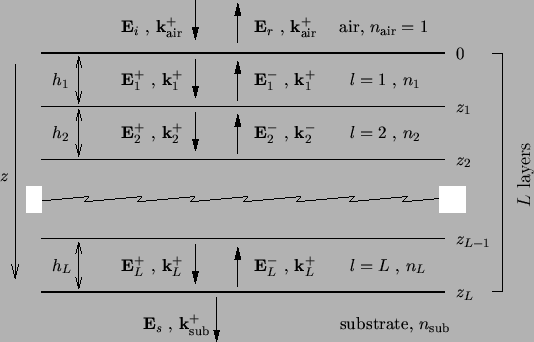We start with the description of the boundary conditions valid at any interface between two adjacent layers l - 1 and l. Elementary results of EM theory postulates that in the absence of a surface current density both the tangential electric and tangential magnetic field components are continuous across the surface [11, p. 6]. Hence we obtain in our notation at any interface position zl - 1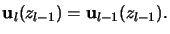(C.20)

Using the relation (C.19) yields the lateral field components at the next interface located at zl = zl - 1 + hl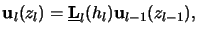(C.21)

whereby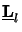is the propagation matrix of layer l. This relation is of fundamental importance as it propagates the field from one interface to the next. Now a recursive evaluation connects the lateral field components at any vertical position z to the field occurring on top of the interface at z = 0 by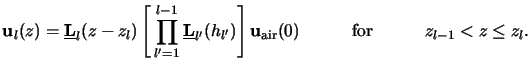(C.22)

At the interface to the substrate, i.e., at the bottom zL of the stack, we thus obtain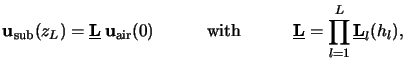(C.23)

whereby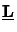is simply called the propagation matrix of the stratified medium. Obviously the lateral field components at the top and at the bottom of the stack are related by this equation. Thus also the wave amplitudes occurring in the air and in the substrate can be related. From (C.19) and (C.14) we obtain uair(h) =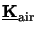eair and usub(h) =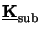esub and the relation looked for writes to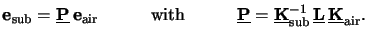(C.24)

Finally, we again leave off the matrix-vector notation used so far to account for the physical situation depicted in Figure C.2. As can be seen the two amplitude vectors eair and esub are given by (cf. (C.9))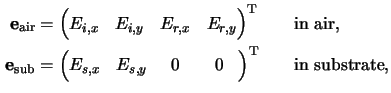because in the substrate only outgoing light has to be considered. By explicitly writing the third and fourth rows of the matrix equation (C.26) we obtain the two necessary conditions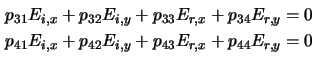that have to be satisfied by the incoming and the reflected light Ei and Er, respectively. These two equations relate the lateral components of the field amplitudes occurring above the stack. They establish the boundary conditions needed for the differential method in case of multiple planar homogeneous layers below the simulation domain (cf. Section 6.3).

For the simple exposure simulation method commonly called transfer matrix algorithm
(cf. Section 5.2.3) one last step is missing. Clearly only the incoming amplitudes Ei are known. They are calculated by the aerial image tool using for example the vector-valued formulation of the Fourier optics as described in Section 4.1.5. Hence the unknown reflected light Er has to be expressed by the incident light Ei. This can easily be done by inverting (C.28) resulting in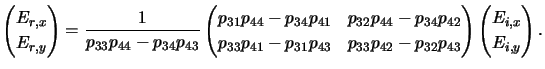(C.25)

The overall transfer matrix algorithm now consists of two steps:

1.
Firstly, the propagation matrix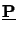in (C.26) is calculated and the reflected amplitudes are derived from the incident ones by (C.29). The lateral field uair(0) occurring at the up-most interface between air and stack is thus determined.
2.
Secondly, the recursion formula (C.24) is evaluated at any desired vertical position z. From the lateral field components u(z) the vertical ones can readily be calculated from (C.4) and the overall EM field is obtained.Next: D. Nonplanar Material Interface Up: C. Stratified Medium Previous: Propagation Matrix
Heinrich Kirchauer, Institute for Microelectronics, TU Vienna
1998-04-17